### Responses And Impedances As Complex Transfer Functions.

-What do we want to measure in the lab ?

We can't initiate the design process unless we have the following data stored in our computer hard disk:

• The SPL and phase responses of the loudspeakers drivers to be mounted on our final speaker system.
• The SPL and phase response of any bass reflex port or vent present on our enclosure baffle.
• The magnitude and phase of the (complex) impedances of all drivers.

During the design process we may also need to measure the crossover filters' (voltage) gain/phase response or the gain/phase response of an audio power or pre- amplifier.

• SPL responses are ratios of sound pressure level (detected by our lab microphone) to voltage signal (fed to the loudspeaker driver).
• Gain responses (of passive or other active sort of filters or of amplification units) are ratios of output to input voltage.
• Impedance of a component or a circuit or a loudspeaker driver is the ratio of voltage applied across its terminals to the current flowing into it. In lab measurements this current is usually monitored by the voltage drop across a small fixed (and known) resistor in series with the component of which the impedance we seek. So again impedance is measured by a voltage gain.

In all these cases the devices or components involved are assumed to be Linear and Time Invariant systems (LTI systems' theory plays a key role in Electronics and Engineering). In these ratios we take the quantity in the nominator to express the system's output and the one in the denominator to stand for the system's input or stimulus. LTI system theory calls these ratios 'Transfer Functions'.

Theory of LTI systems states that when these systems are stimulated by an oscillatory signal of frequency f their output is also an oscillation of frequency f. Output oscillation has a different amplitude and a phase difference with respect to input.

Let input be an oscillation x(t)= Xo * cos(ω*t), where Xo is its amplitude and ω the angular frequency 2*π*f.

Output will be of the form y(t)= Yo * cos(ω*t+φ), where Υo is the respective amplitude and φ the phase difference or delay between output and input oscillation. Yo and φ change with frequency.

Generally speaking LTI systems do two things: they change the amplitude and shift the phase of input oscillations.

The reason for writing these two expressions is only to prove that when we try to get their ratio and define the associated Transfer Functions we end up with a problem; the ratio of two oscillatory cosine functions gives nothing.

The only way to escape this problem and define useful transfer functions is to move this discussion to the field of complex numbers.

Now input oscillation becomes X(t) = Xo * eiωt, where constant e stands for the base of natural logarithms e=2.1718..

(Perhaps it would be a good idea to check your high school algebra for the properties of this exponential that relate it to cosines and sines : ei * .. = cos(..) + i sin(..) ).

Output becomes Y(t) = Yo * eiωt+iφ. The ratio of output to input is easily defined :

H = Y/X = Yo/Xo * ( eiωt+iφ / eiωt) = Yo/Xo * e .

So a system's transfer function H(f) is now defined to be a complex function of frequency.

The Algebra of complex numbers states that a complex number can be represented in two ways :

• either in the usual form : real part +imaginary part *i , or
• in the exponential form : magnitude * ei*phase argumant .

Both forms relate to each other : a complex number's magnitude equals √(real^2+imag^2) and its phase argument is the angle having a tangent equal to imag/real.

Let us have some examples of all these absurd notions.

A filter is fed with a single frequency (100Hz) oscillatory signal of amplitude 2 Volts peak. Its output oscillation was found to have an amplitude of 1 Volt and a phase lead of 45 degrees.

Input is denoted by complex number X=2 * ei*2*π*100*t.

We write 45 degrees as π/4 radians and filter's output becomes Y=1 * ei*2*π*100*t+i*π/4.

Taking Y/X for the filter's transfer function at this frequency we get H=Y/X= 0.5 ei*π/4.

Magnitude of the transfer function gives the gain response of this filter device : 0.5 or -6dB

The phase argument of the transfer function gives the phase difference that this device establishes between its output and input oscillatory signals : π/4 or +45 degrees.

Of course a transfer function changes with frequency; so change its magnitude (gain response) and its phase argument (phase response).

Now let us have an example of impedance.

A loudspeaker driver is fed with a single frequency (400Hz) oscillatory signal of amplitude 3.5 Volts peak. Its current was found to be an oscillation with a peak value of 0.5 Amperes and a phase lag of -30 degrees with respect to voltage.

Input voltage is denoted by complex number V=3.5 * ei*2*π*400*t.

We write 30 degrees as π/6 radians and current becomes I=0.5 * ei*2*π*400*t-i*π/6.

Taking V/I for the drivers's impedance at this frequency we get Z=V/I= 7 e+i*π/6.

Impedance magnitude gives the driver's absolute impedance at this frequency 7 Ohms, which is rather typical for '8-Ohm' woofer drivers at 300-400 Hz.

The phase argument of impedance gives the phase difference that this device establishes between its current and its voltage at this frequency. In this case voltage leads the current (or current lags the voltage) by +30 degrees. This behaviour is said to be inductive. Actually most woofer drivers behave inductively above 300Hz.

Impedance changes with frequency; so change its magnitude (absolute impedance) and its phase argument (phase lead or lag between voltage and current).

www.diy-loudspeakers.com

### Who is online

We have 81 guests and no members online

### Visitors since 8/4/1541.2% USA4.0% Germany3.8% United Kingdom3.4% China3.3% Russia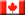3.1% Canada3.0% France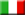3.0% Italy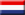2.8% Netherlands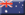2.6% Australia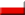2.3% Poland1.6% Sweden

Total:

200

Countries
235542
Today: 146
Yesterday: 136
This Month: 649
Last Month: 4,443